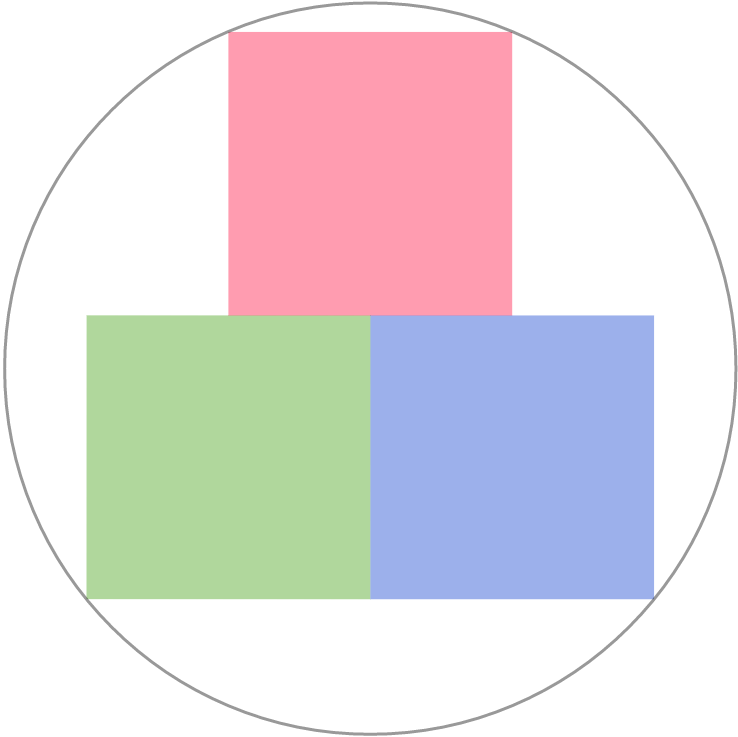# Tight Squares

Geometry Level 3The figure shows a packing of three identical squares in a circle.

If we define $\text{Packing Efficiency}=\frac{\text{Total area of the packing material}}{\text{Total area of the container}},$

To the nearest integer, how efficient (in percentage) is the packing in this case?

×